# Wilcox Solar Observatory Magnetograms

## #8290 - 8299, 2005.06.05 - 2005.06.13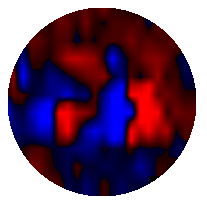```WSO Magnetogram sn=8290	 observed at 2005:06:05_21h:19m:16s
Central Meridian Carrington Longitude CT2030:118
Sky = 0 (5 worst)
trouble=00004000 NOTE:
12 intensity variance exceeds 5.0
``````WSO Magnetogram sn=8291	 observed at 2005:06:06_20h:13m:16s
Central Meridian Carrington Longitude CT2030:105
Sky = 0 (5 worst)
trouble=00004000 NOTE:
12 intensity variance exceeds 5.0
```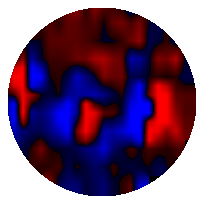```WSO Magnetogram sn=8292	 observed at 2005:06:06_22h:21m:25s
Central Meridian Carrington Longitude CT2030:104
Sky = 0 (5 worst)
trouble=00004000 NOTE:
12 intensity variance exceeds 5.0
```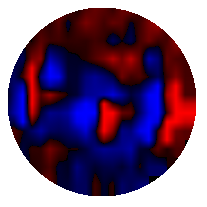```WSO Magnetogram sn=8293	 observed at 2005:06:07_19h:38m:10s
Central Meridian Carrington Longitude CT2030:092
Sky = 0 (5 worst)
trouble=00004000 NOTE:
12 intensity variance exceeds 5.0
```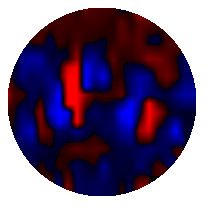```WSO Magnetogram sn=8294	 observed at 2005:06:10_00h:06m:14s
Central Meridian Carrington Longitude CT2030:063
Sky = 0 (5 worst)
trouble=00004000 NOTE:
12 intensity variance exceeds 5.0
```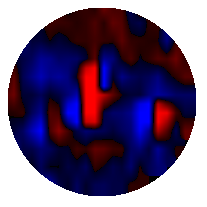```WSO Magnetogram sn=8295	 observed at 2005:06:10_19h:33m:12s
Central Meridian Carrington Longitude CT2030:052
Sky = 0 (5 worst)
trouble=00004000 NOTE:
12 intensity variance exceeds 5.0
```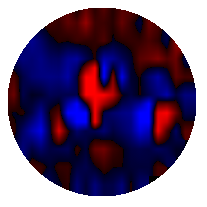```WSO Magnetogram sn=8296	 observed at 2005:06:10_21h:43m:51s
Central Meridian Carrington Longitude CT2030:051
Sky = 0 (5 worst)
trouble=00004000 NOTE:
12 intensity variance exceeds 5.0
```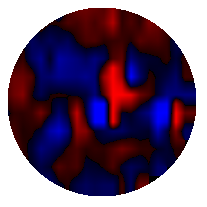```WSO Magnetogram sn=8297	 observed at 2005:06:11_21h:54m:19s
Central Meridian Carrington Longitude CT2030:038
Sky = 0 (5 worst)
trouble=00004000 NOTE:
12 intensity variance exceeds 5.0
```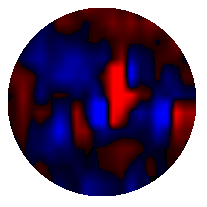```WSO Magnetogram sn=8298	 observed at 2005:06:12_00h:02m:13s
Central Meridian Carrington Longitude CT2030:037
Sky = 0 (5 worst)
trouble=00004000 NOTE:
12 intensity variance exceeds 5.0
```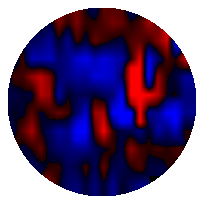```WSO Magnetogram sn=8299	 observed at 2005:06:13_01h:03m:37s
Central Meridian Carrington Longitude CT2030:023
Sky = 1 (5 worst)
trouble=00004000 NOTE:
12 intensity variance exceeds 5.0
```